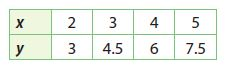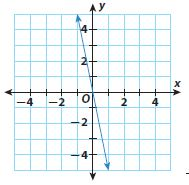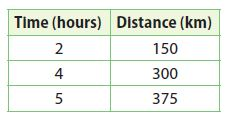Homework Explained - Math Practice 101Dear guest, you are not a registered member. As a guest, you only have read-only access to our books, tests and other practice materials.

As a registered member you can:

Registration is free and doesn't require any type of payment information. Click here to Register.
Go to page:
Chapter 3: Proportional Relationships; Model Quiz

3.1 Representing Proportional Relationships

• Question 1

Find the constant of proportionality for the table of values.• Question 2

Phil is riding his bike. He rides 25 miles in 2 hours, 37.5 miles in 3 hours, and 50 miles in 4 hours. Find the constant of proportionality and write an equation to describe the situation.

• Type below:

3.2 Rate of Change and Slope

Find the slope of each line.

• Question 3• Question 43.3 Interpreting the Unit Rate as Slope

• Question 5

The distance Train A travels is represented by d = 70t, where d is the distance in kilometers and t is the time in hours. The distance Train B travels at various times is shown in the table. What is the unit rate of each train? Which train is going faster?Essential Question

• Question 6

What is the relationship among proportional relationships, lines, rates of change, and slope?

• Type below:

Yes, email page to my online tutor. (if you didn't add a tutor yet, you can add one here)